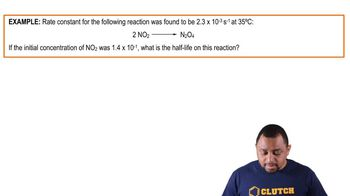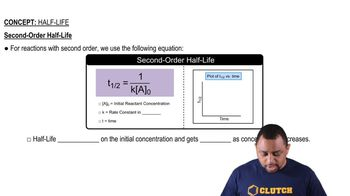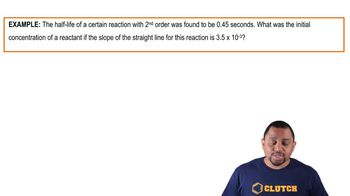Start typing, then use the up and down arrows to select an option from the list.# Half-Life Example 1

Jules Bruno
149views
1
the reverse haber reaction has a rate constant of 1.45 times 10 to negative six polarity times seconds inverse at 25 degrees Celsius. Now calculate the half life for the reaction, where the initial concentration of ammonia equals 2.47 times 10 to the negative two moles per liter. Alright, so here we know that this is a zero order reaction. Because remember for a zero order reaction, the units for your rate constants are polarity times time inverse. So the fact that its polarity times seconds in verse tells us this is a zero order reaction. So here that means that half life equals the initial concentration of your reactant divided by two K. So this becomes a simple plug and chug type of question. We have the initial concentration of our reactant in terms of ammonia divided by two times our rate constant here, malaria, t times seconds inverse. The polarities will cancel out and our time our half life will be in seconds. So this comes out to be 8.52 times to the three seconds. So this would be our final answer.02:1701:1901:4901:1801:4401:52